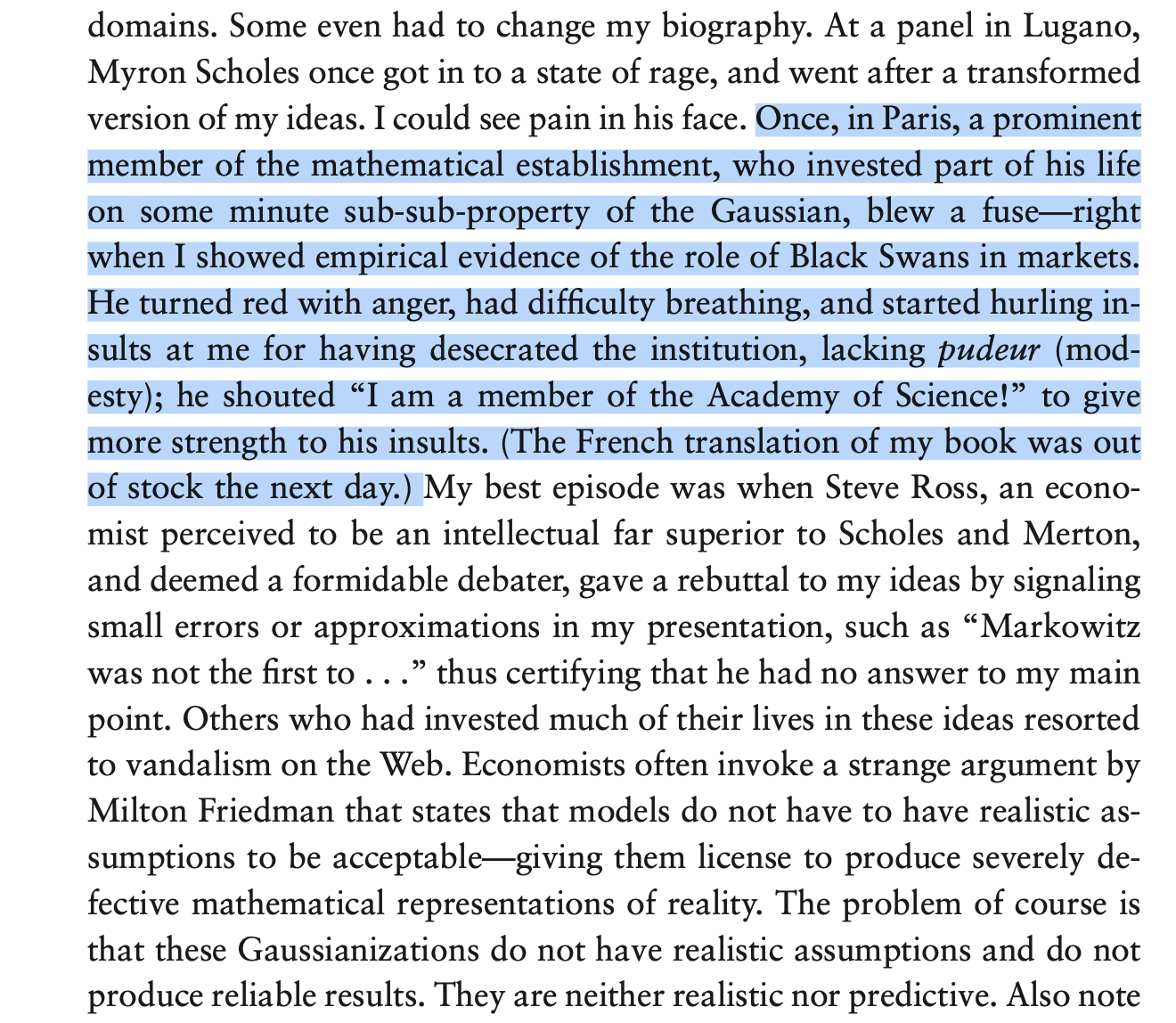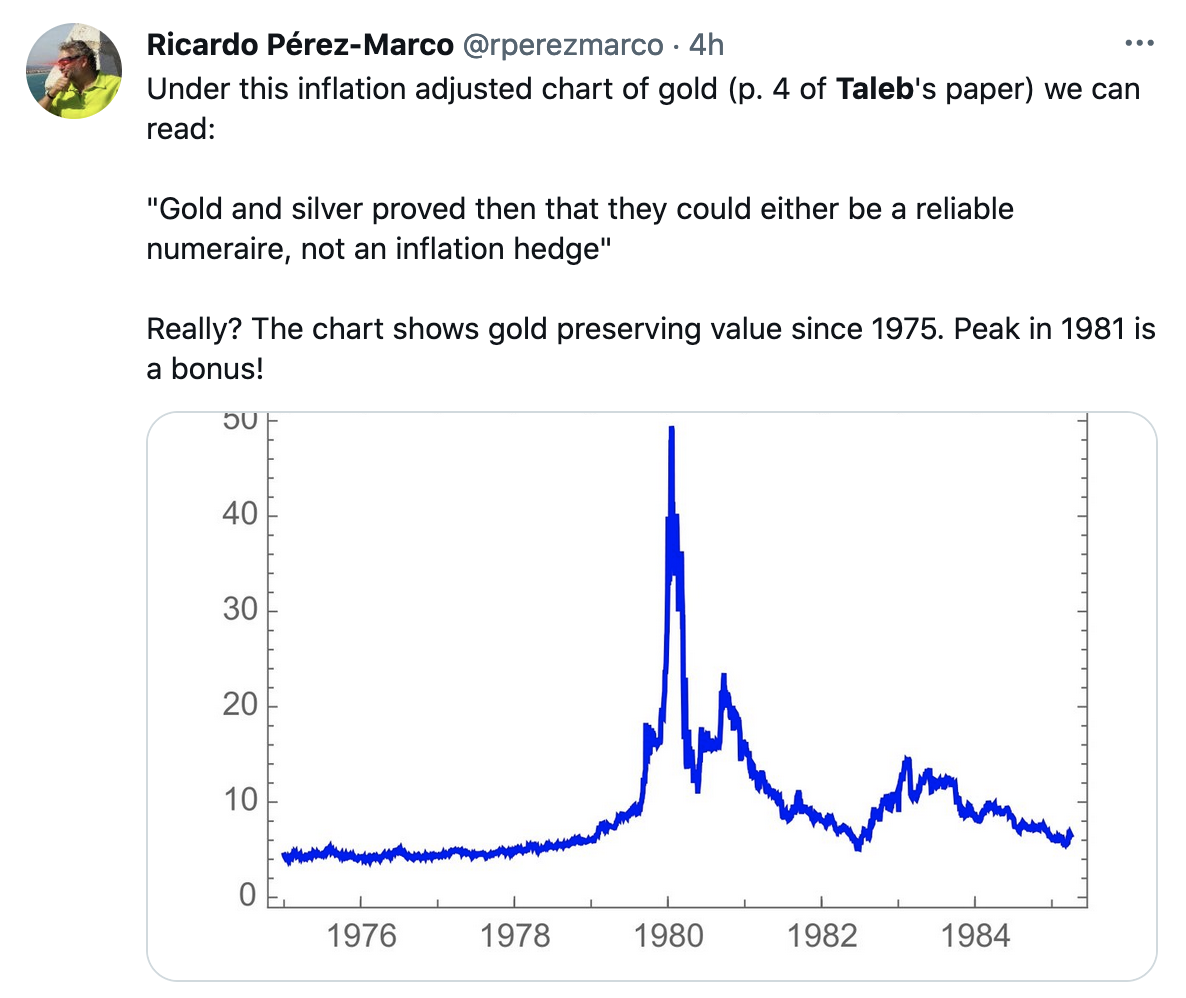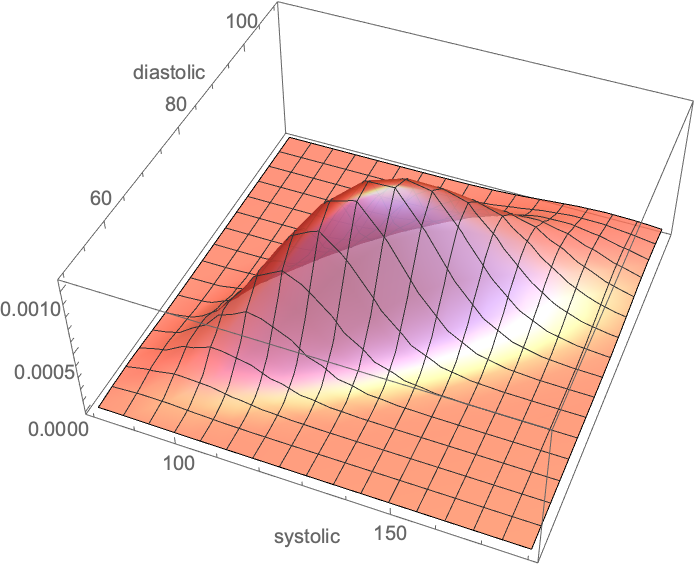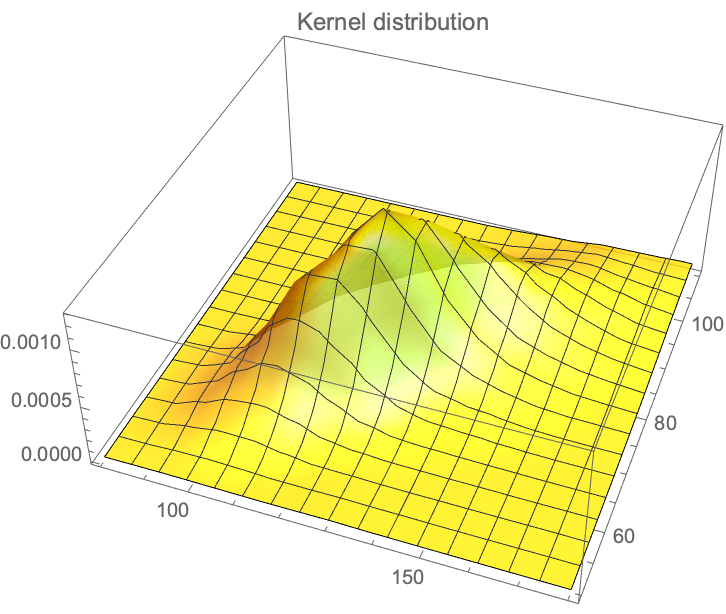On weighted sums of Uniformly distributed random variables

Ariely.nb
The “Ariely” fake result reveal a probability distribution totally foreign to psychologists

A nice Limit

From @infseriesbot, prove the identity: $$\gamma_n = \displaystyle\int_1^\infty \frac{\{x\}}{x^2} (n-\log(x)) \log^{n-1} \, dx$$.

We have $$\{x\}=x-i \, \text{for} \, i \leq x \leq i+1$$,

so

$$\text{rhs}=\displaystyle\sum _{i=1}^{\infty } \displaystyle\int_i^{i+1} \frac{(x-i) (n-\log (x)) \log ^{n-1}(x)}{x^2} \, dx,$$

and since $$\displaystyle\int \frac{(x-i) (n-x \log ) \left(x \log ^{n-1}\right)}{x^2} \, dx=\log ^n(x) \left(-\frac{i}{x}-\frac{\log (x)}{n+1}+1\right)$$

$$\displaystyle \int_i^{i+1} \frac{(x-i) (n-\log (x)) \log ^{n-1}(x)}{x^2} \, dx=\frac{(i+1) \log ^{n+1}(i)+(-(i+1) \log (i+1)+n+1) \log ^n(i+1)}{(i+1) (n+1)}$$,

allora:

$$\text{rhs}=\underset{T\to \infty }{\text{lim}}\left(\frac{(n-(T+1) \log (T+1)+1) \log ^n(T+1)}{(n+1) (T+1)}+\sum _{i=2}^T \frac{\log ^n(i)}{i}\right)$$,

and since $$\frac{\log ^n(T+1) (n-(T+1) \log (T+1)+1)}{(n+1) (T+1)}\overset{T}{\to}\frac{\log ^{n+1}(T)}{n+1}$$,

From the series representation of the Stieltjes Gamma function, $$\gamma_n$$:

$$\underset{T\to \infty }{\text{lim}}\left(\sum _{i=1}^T \frac{\log ^n i}{i}-\frac{\log ^{n+1}(T)}{n+1}\right)=\gamma _n$$

Criticism of the “Bitcoin Black Paper”

So far I received only one sound piece of criticism, by the financial economist Gur Huberman. Just as a painting has a utility to a collector, akin to a consumer good, bitcoin has utility for… fraudsters.

This is indeed correct but somehow it still doesn’t make much difference. Why? Because, it is turning out, bitcoin is way too transparent for a real fraudster to escape end-point busting. And, indeed, other cryptocurrencies such as monero may do a better job. And this service must be temporary, as in the traditional tug of war between cops and thieves. Consider that if bitcoin becomes a currency just for thieves, it will have no utility… for thieves. So yes, Gur is correct and bitcoin is worth something more than zero. But such a value is residual and must reach zero soon.

And of course I got a lot of junk arguments.

Junk Arguments

Ricardo Perez Marco would not have been discussed here had he not acted in bad faith. He is a professional mathematician we hired at #RWRI to discuss bitcoin, which we were trying to undersdand. He subsequently stabbed us in the back, as he turned on us, offended that we subsequently made negative public comments about bitcoin, which to him, is perfection. Yet nothing negative about him was said: it was just about bitcoin. (Note that in a long finance career I’ve never seen anyone offended that some other trader is bullish on something when he or she is short it. Never ).

I’ve seen religious fundamentalists less offended by the desecration of their gods. He has, of course, posted series of comments on the paper.

Perez Marco is another example of the professional abstract mathematician incapable of grasping elementary (I mean really elementary) financial concepts, let alone basic logical elements from the real world, and not realizing it. These poseurs very often fail to assimilate simple financial equations and relationships that truck drivers get instantly; they get arbitraged quickly, and picked on without even realizing it.

As I keep writing in the Incerto, people in quantitative finance (and economics) are extremely familiar with the problem –we avoid hiring “theorems and lemma people”, particularly those with a sense of entitlement, prefering empirically rigorous persons who can do solid math without drowning in it. It would be like asking bookkeepers for trading advice! (Simon from Renaissance is perhaps the only exception.) Perez-Marco, as math-poseur, wrote about Satoshi Nakamoto, the alleged author of the original white paper, who made a minor mistake: “He is smart but he is not a mathematician”. If I had $1 every time I spotted a mathematician making an elementary mathematical mistake in finance and probability … For instance, Perez Marco proved incapable to comprehend that if you can buy goods in the supermarket using a bitcoin credit card, it doesn’t mean that a price is denominated in bitcoin (denomination entails a liability). Nor did he grasp the economic notion of substitution applied to electricity uses. Now in his “rebuttals”, he still doesn’t get it; he has compared bitcoin to the CHF, when the CHF is used… as a currency by millions people transacting in it daily, in prices denominated in it, while there are no goods and services denominated in #BTC. Nor can he get some basic assumptions concerning the real world in the specification of financial dynamics. Nor does he understand hazard models. Perez-Marco keeps saying the math is “wrong”, every time on something different. So far, no meat. A Subplot I’ve left Perez Marco alone to do his barking for a few months, given the #RWRI link, but he crossed the line at some point into abject behavior, by dissing #RWRI and promoting lies. And he invoked the hatred Paul Malliavin had for me, as witnessed by the following episode. Malliavin got angry, on the eve of the 2007-8 crash, that a mere trader would criticize the models that he, a member of the French Academy of Science, was defending. He died right after that and I removed his name out of respect for the recently dead.That was Paul Malliavin, on the eve of the 2007 crash, defending financial models. Eggregious behavior: Perez-Marco blocked me on Twitter (again solely for disliking bitcoin). Then he tried to reach me privately to warn me against going to a competing crypto conference on grounds that someone “was a fraud” (according to bitcoiners’ mob rule, not any court of law). They don’t want me to go the competitor’s conference but do not invite me to their own conference! For nobody invited me to address the #BTC conference running at about the same time (though one of the protagonists Michael Saylor has been formally accused of fraud by the SEC). And it did not hit him to apologize for the mob harassment that his group has been subjecting me to (close to 16,000 trolls).Perez-Marco can’t see the difference between Gold and Silver, nor can he get the difference between raw and inflation adjusted chart. Aside from the fact that he doesn’t get the notion of maximum drawdown as a measure of riskiness. Selgin Cato’s institute George Selgin said a looooot of vague things (with some erroneous financial reasoning ) but has not said anything about the paper. The Lognormal at high variance: a probability paradox You have zero probability of making money. But it is a great trade. One-tailed distributions entangle scale and skewness. When you increase the scale, their asymmetry pushes the mass to the right rather than bulge it in the middle. They also illustrate the difference between probability and expectation as well as the difference between various modes of convergence. Consider a lognormal $$\mathcal{LN}$$ with the following parametrization, $$\mathcal{LN}\left[\mu t-\frac{\sigma ^2 t}{2},\sigma \sqrt{t}\right]$$ corresponding to the CDF $$F(K)=\frac{1}{2} \text{erfc}\left(\frac{-\log (K)+\mu t-\frac{\sigma ^2 t}{2}}{\sqrt{2} \sigma \sqrt{t}}\right)$$. The mean $$m= e^{\mu t}$$, does not include the parameter $$\sigma$$ thanks to the $$-\frac{\sigma ^2}{2} t$$ adjustment in the first parameter. But the standard deviation does, as $$STD=e^{\mu t} \sqrt{e^{\sigma ^2 t}-1}$$. When $$\sigma$$ goes to $$\infty$$, the probability of exceeding any positive $$K$$ goes to 0 while the expectation remains invariant. It is because it masses like a Dirac stick at $$0$$ with an infinitesimal mass at infinity which gives it a constant expectation. For the lognormal belongs to the log-location-scale family. $$\underset{\sigma \to \infty }{\text{lim}} F(K)= 1$$ Option traders experience an even worse paradox, see my Dynamic Hedging. As the volatility increases, the delta of the call goes to 1 while the probability of exceeding the strike, any strike, goes to $$0$$. More generally, a $$\mathcal{LN}[a,b]$$ has for mean, STD, and CDF $$e^{a+\frac{b^2}{2}},\sqrt{e^{b^2}-1} e^{a+\frac{b^2}{2}},\frac{1}{2} \text{erfc}\left(\frac{a-\log (K)}{\sqrt{2} b}\right)$$ respectively. We can find a parametrization producing weird behavior in time as $$t \to \infty$$. Thanks: Micah Warren who presented a similar paradox on Twitter. The “Bitcoin, Currencies and Fragility” Paper The main paper Bitcoin, Currencies and Fragility is updated here . BTC-QF The supplementary material is updated here www.fooledbyrandomness.com/BTC-QF-appendix.pdf BTC-QF-appendix A Peculiar Integral Prove $$I= \displaystyle\int_{-\infty }^{\infty}\sum_{n=0}^{\infty } \frac{\left(-x^2\right)^n }{n!^{2 s}}\; \mathrm{d}x= \pi^{1-s}$$. We can start as follows, by transforming it into a generalized hypergeometric function: $$I=\displaystyle\int_{-\infty }^{\infty }\, _0F_{2 s-1} (\overbrace{1,1,1,…,1}^{2 s-1 \text{times}}; -x^2)\mathrm{d}x$$, since, from the series expansion of the generalized hypergeometric function, $$\, _pF_q\left(a_1,a_p;b_1,b_q;z\right)=\sum_{k=0}^{\infty } \frac{\prod_{j=1}^p \left(a_j\right)_k z^k}{\prod_{j=1}^q k! \left(b_j\right)_k}$$, where $$(.)_k$$ is the Pochhammer symbol $$(a)_k=\frac{\Gamma (a+k)}{\Gamma (a)}$$. Now the integrand function does not appear to be convergent numerically, except for $$s= \frac{1}{2}$$ where it becomes the Gaussian integral, and the case of $$s=1$$ where it becomes a Bessel function. For $$s=\frac{3}{2}$$ and $$x=10^{19}$$, the integrand takes values of $$10^{1015852872356}$$ (serious). Beyond that the computer starts to produce smoke. Yet it eventually converges as there is a closed form solution. It is like saying that it works in theory but not in practice! For, it turns out, under the restriction that $$2 s\in \mathbb{Z}_{>\, 0}$$, we can use the following result: $$\int_0^{\infty } t^{\alpha -1} _pF_q \left(a_1,\ldots ,a_p;b_1,\ldots ,b_q;-t\right) \, dt=\frac{\Gamma (\alpha ) \prod {k=1}^p \Gamma \left(a_k-\alpha \right)}{\left(\prod {k=1}^p \Gamma \left(a_k\right)\right) \prod {k=1}^q \Gamma \left(b_k-\alpha \right)}$$ Allora, we can substitute $$x=\sqrt(u)$$, and with $$\alpha =\frac{1}{2},p=0,b_k=1,q=2 s-1$$, given that $$\Gamma(\frac{1}{2})=\sqrt(\pi)$$, $$I=\frac{\sqrt{\pi }}{\prod _{k=1}^{2 s-1} \sqrt{\pi }}=\pi ^{1-s}$$. So either the integrand eventually converges, or I am doing something wrong, or both. Perhaps neither. On the Multivariate Lognormal with Application to Blood Pressure Background: We’ve discussed blood pressure recently with the error of mistaking the average ratio of systolic over diastolic for the ratio of the average of systolic over diastolic. I thought that a natural distribution would be the gamma and cousins, but, using the Framingham data, it turns out that the lognormal works better. For one-tailed distribution, we do not have a lot of choise in handling higher dimensional vectors. There is some literature on the multivariate gamma but it is neither computationally convenient nor a particular good fit. Well, it turns out that the Lognormal has some powerful properties. I’ve shown in a paper (now a chapter in The Statistical Consequences of Fat Tails) that, under some parametrization (high variance), it can be nearly as “fat-tailed” as the Cauchy. And, under low variance, it can be as tame as the Gaussian. These academic disputes on whether the data is lognormally or power law distributed are totally useless. Here we realize that by using the method of dual distribution, explained below, we can handle matrices rather easily. Simply, if $$Y_1, Y_2, \ldots Y_n$$ are jointly lognormally distributed with a covariance matrix $$\Sigma_L$$, then $$\log(Y_1), \log(Y_2), \ldots \log(Y_n)$$ are normally distributed with a matrix $$\Sigma_N$$. As to the transformation $$\Sigma_L \to \Sigma_N$$, we will see the operation below. Let $$X_1=x_{1,1},\ldots,x_{1,n}, X_2= x_{2,1},\dots x_{2,n}$$ be joint distributed lognormal variables with means $$\left(e^{\mu _1+\frac{\sigma _1^2}{2}}, e^{\mu _2+\frac{\sigma _2^2}{2}}\right)$$ and a covariance matrix $$\Sigma_L=\left(\begin{array}{cc}\left(e^{\sigma _1^2}-1\right) e^{2 \mu _1+\sigma _1^2}&e^{\mu _1+\mu _2+\frac{\sigma _1^2}{2}+\frac{\sigma _2^2}{2}}\left(e^{\rho \sigma _1 \sigma _2}-1\right)\\ e^{\mu _1+\mu _2+\frac{\sigma _1^2}{2}+\frac{\sigma _2^2}{2}}\left(e^{\rho \sigma _1 \sigma _2}-1\right)&\left(e^{\sigma _2^2}-1\right) e^{2 \mu _2+\sigma _2^2}\end{array}\right)$$ allora $$\log(x_{1,1}),\ldots, \log(x_{1,n}), \log(x_{2,1}),\dots \log(x_{2,n})$$ follow a normal distribution with means $$(\mu_1, \mu_2)$$ and covariance matrix $$\Sigma_N=\left(\begin{array}{cc}\sigma _1^2&\rho \sigma _1 \sigma _2 \\ \rho \sigma _1 \sigma _2&\sigma _2^2 \\ \end{array}\right)$$ So we can fit one from the other. The pdf for the joint distribution for the lognormal variables becomes:Bivariate Lognormal Distribution $$f(x_1,x_2)= \frac{\exp \left(\frac{-2 \rho \sigma _2 \sigma _1 \left(\log \left(x_1\right)-\mu _1\right) \left(\log \left(x_2\right)-\mu _2\right)+\sigma _1^2 \left(\log \left(x_2\right)-\mu _2\right){}^2+\sigma _2^2 \left(\log \left(x_1\right)-\mu _1\right){}^2}{2 \left(\rho ^2-1\right) \sigma _1^2 \sigma _2^2}\right)}{2 \pi x_1 x_2 \sqrt{-\left(\left(\rho ^2-1\right) \sigma _1^2 \sigma _2^2\right)}}$$Kernel Distribution We have the data from the Framingham database for, using $$X_1$$ for the systolic and $$X_2$$ for the diastolic, with $$n=4040, Y_1= \log(X_1), Y_2=\log(X_2): {\mu_1=4.87,\mu_2=4.40, \sigma_1=0.1575, \sigma_2=0.141, \rho= 0.7814}$$, which maps to: $${m_1=132.35, m_2= 82.89, s_1= 22.03, s_2=11.91}$$. Some (mis)Understanding of life Expectancy, with some good news. The Lancet article: Maron, Barry J., and Paul D. Thompson. “Longevity in elite athletes: the first 4-min milers.” The Lancet 392, no. 10151 (2018): 913 contains an eggregious probabilistic mistake in handling “expectancy” a severely misunderstood –albeit basic– mathematical operator. It is the same mistake you read in the journalistic “evidence based” literature about ancient people having short lives (discussed in Fooled by Randomness), that they had a life expectancy (LE) of 40 years in the past and that we moderns are so much better thanks to cholesterol lowering pills. Something elementary: unconditional life expectancy at birth includes all people who are born. If only half die at birth, and the rest live 80 years, LE will be ~40 years. Now recompute with the assumption that 75% of children did not make it to their first decade and you will see that life expectancy is a statement of, mostly, child mortality. It is front-loaded. As child mortality has decreased in the last few decades, it is less front-loaded but it is cohort-significant. The article (see the Table below) compares the life expectancy of athletes in a healthy cohort of healthy adults to the LE at birth of the country of origin. Their aim was to debunk the theory that while exercise is good, there is a nonlinear dose-response and extreme exercise backfires. Something even more elementary missed in the Lancet article. If you are a nonsmoker, healthy enough to run a mile (at any speed), do not engage in mafia activities, do not do drugs, do not have metabolic syndrome, do not do amateur aviation, do not ride a motorcycle, do not engage in pro-Trump rioting on Capitol Hill, etc., then unconditional LE has nothing to do with you. Practically nothing. Just consider that 17% of males today smoke (and perhaps twice as much at the time of the events in the “Date” column of the table). Smoking reduces your life expectancy by about 10 years. Also consider that a quarter or so of Americans over 18 and more than half of those over 50 have metabolic syndrome (depending on how it is defined). Lindy and NonLindy Now some math. What is the behavior of life expectancy over time? Let $$X$$ be a random variable that lives in $$(0,\infty)$$ and $$\mathbb{E}$$ the expectation operator under “real world” (physical) distribution. By classical results, see the exact exposition in The Statistical Consequences of Fat Tails: $$\lim_{K \to \infty} \frac{1}{K} \mathbb{E}(X|_{X>K})= \lambda$$ If $$\lambda=1$$ , $$X$$ is said to be in the thin tailed class $$\mathcal{D}_1$$ and has a characteristic scale . It means life expectancy decreases with age, owing to senescence, or, more rigorously, an increase of the force of mortality/hazard rate over time. If $$\lambda>1$$ , $$X$$ is said to be in the fat tailed regular variation class $$\mathcal{D}2$$ and has no characteristic scale. This is the Lindy effect where life expectancy increases with age. If $$\lim_{K \to \infty} \mathbb{E}(X|_{X>K})-K= \lambda$$ where $$\lambda >0$$, then $$X$$ is in the borderline exponential class. The next conversation will be about the controversy as to whether human centenarians, after aging is done, enter the third class, just like crocodiles observed in the wild, where LE is a flat number (but short) regardless of age. It may be around 2 years whether one is 100 or 120. Another Probability Error in Medicine (Golden Ratio) In Yalta, K., Ozturk, S., & Yetkin, E. (2016). “Golden Ratio and the heart: A review of divine aesthetics”, International Journal of Cardiology214, 107-112, the authors compute the ambulatory ratio of Systolic to Diastolic by averaging each and taking the ratio. “Mean values of diastolic and systolic pressure levels during 24-h, day-time and night-time recordings were assessed to calculate the ratios of SBP/DBP and DBP/PP in these particular periods”. The error is to compute the mean SBP and mean DBP then get the ratio, rather than compute every SBP/DBP data point. Simply, $$\frac{\frac{1}{n}\sum_{i=1}^n x_i}{\frac{1}{n}\sum_{i=1}^n y_i}\neq \frac{\sum _{i=1}^n \frac{x_i}{y_i}}{n}$$ Easy to see with just n=2: $$\frac{x_1+x_2}{y_1+y_2}\neq \frac{1}{2} \left(\frac{x_1}{y_1}+\frac{x_2}{y_2}\right)$$. The rest is mathematical considerations until I get real data to find the implication of this error that seems to have seeped through the literature (we know there is an eggregious mathematical error; how severe the consequences need to be assessed from data.). For the intuition of the problem consider that when people tell you that healthy people have on average BP of 120/80, that those whose systolic is 120 must have a diastolic 80, and vice-versa, which can only be true if the ratio is deterministic . Clearly, from Jensen’s inequality, where $$X$$ and $$Y$$ are random variables, whether independent or dependent, correlated or uncorrelated, we have: $$\mathbb{E}(X/Y) \neq \frac{\mathbb{E}(X)} {\mathbb{E}(Y)}$$ with few exceptions, s.a. a perfectly correlated (positively or negatively) $$X$$ and $$Y$$ in which case the equality is forced by the fact that the ratio becomes a degenerate random variable. Inequality: At the core lies the fundamental ratio inequality (by Jensen’s) that: $$\frac{1}{n}\sum _{i=1}^n \frac{1}{y_i} \geq \frac{1}{ \frac{\sum _{i=1}^n y_i}{n}}$$, or $$\mathbb{E}(\frac{1}{Y})\geq\frac{1}{\mathbb{E}(Y)}$$. The proof is easy: $$\frac{1}{y}$$ is a convex function of y and has a positive second derivative. Allora when $$X$$ and $$Y$$ are independent, we have the ratio distribution $$\mathbb{E}(\frac{X}{Y}) = \mathbb{E}(X) \times \mathbb{E}(\frac{1}{Y})\geq \frac{\mathbb{E}(X)} {\mathbb{E}(Y)}$$ Furthermore, where the two variables have support on $$(-\infty, \infty)$$, say a Gaussian distribution $$\mathcal{N} (\mu_1,\sigma_1)$$, the mean of the ratio is infinite. How? Simply , for $$Z_1= \frac{1}{Y}$$, $$f(z_1)=\frac{e^{-\frac{(\mu z_1-1)^2}{2 \sigma ^2 z_1^2}}}{\sqrt{2 \pi } \sigma z^2}$$ $$z\neq 0$$ From where we can work out the counterintuitive result that if $$X$$ and $$Y$$ $$\sim$$ $$\mathcal{N}(0,\sigma_1)$$ and $$\mathcal{N}(0,\sigma_2)$$ respectively, $$\frac{X}{Y} \sim Cauchy(0,\frac{\sigma_1}{\sigma_2})$$, with infinite moments. As a nice exercise we can get the exact PDF under some correlation structure $$\rho$$ in a bivariate normal: $$f(z)= \frac{\sigma _1 \sigma _2 \sqrt{-\left(\left(\rho ^2-1\right) \left(\sigma _1^2+\sigma _2^2 z^2-2 \rho \sigma _2 \sigma _1 z\right)\right)}}{\pi \left(\sigma _1^2+\sigma _2^2 z^2-2 \rho \sigma _2 \sigma _1 z\right){}^{3/2}}$$, with a mean $$\frac{i \sqrt{\rho ^2-1} \sigma _1 \sigma _2}{\pi \left(\sigma _1^2+\sigma _2^2 z^2-2 \rho \sigma _2 \sigma _1 z\right)}$$ that exists only if $$\rho=1$$ (that is will be 0 in the exactly symmetric case). Luckily, SBP ($$X$$) and DBP ($$Y$$) live in $$(0, \infty)$$ which should yield a finite mean and allow us to use Mellin’s transform which is a good warm up after the holidays (while witing for the magisterial Algebra of Random Variables to arrive by mail). Note: For a lognormal distribution parametrized with $$\mu_1, \mu_2, \sigma_1,\sigma_2$$, under independence: $$\frac{\mathbb{E} X}{\mathbb{E} Y}= e^{-\sigma _2^2}\mathbb{E}\left(\frac{X}{Y}\right)$$ Owing to the fact that the ratio follows another lognormal with for parameters $$\left[\mu _1-\mu _2,\sqrt{\sigma _1^2+\sigma _2^2}\right]$$. Gamma: I’ve calibrated from various papers that it must be a gamma distribution with standard deviations of 14-24 and 10-14 respectively. There are papers on bivariate (multivariate) gamma distributions in the statistics literature (though nothing in the DSBR, the “Data Science Bullshitters Recipes”), but on this distribution later. We can work out that if $$X \sim \mathcal{G}(a_1,b_1)$$ (gamma) and $$Y \sim \mathcal{G}(a_2, b_2)$$, assuming independence (for now), we have the ratio $$Z$$ $$f(z)=\frac{b_1^{a_2} b_2^{a_1} z^{a_1-1} \Gamma \left(a_1+a_2\right) \left(b_2 z+b_1\right){}^{-a_1-a_2}}{\Gamma \left(a_1\right) \Gamma \left(a_2\right)}$$ with mean $$\mathbb{E}(Z)= \frac{a_1 b_1}{\left(a_2-1\right) b_2}$$ while $$\frac{\mathbb{E}(X)} {\mathbb{E}(Y)}= \frac{a_1 b_1}{a_2 b_2}$$.Assuming Gamma Distribution Pierre Zalloua has promised me 10,000 BP observations so we can compute the ratios under a correlation structure. Difference between two binomial random variables (the Danish Mask Study) The Danish Mask Study presents the interesting probability problem: the odds of getting 5 infections for a group of 2470, vs 0 for one of 2398. It warrants its own test statistic which allows us to look at all conditional probabilities. Given that we are dealing with tail probabilities, normal approximations are totally out of order. Further we have no idea from the outset on whether the sample size is sufficient to draw conclusions from such a discrepancy (it is). There appears to be no exact distribution in the literatrue for $$Y=X_ 1-X_2$$ when both $$X_1$$ and $$X_2$$ are binomially distributed with different probabilities. Let’s derive it. Let $$X_1 \sim \mathcal{B}\left(n_1,p_1\right)$$, $$X_2 \sim \mathcal{B}\left(n_2,p_2\right)$$, both independent. We have the constrained probability mass for the joint $$\mathbb{P}\left(X_1=x_1,X_2=x_2\right)=f\left(x_2,x_1\right)$$: $$f\left(x_2,x_1\right)= p_1^{x_1} p_2^{x_2} \binom{n_1}{x_1} \binom{n_2}{x_2} \left(1-p_1\right){}^{n_1-x_1} \left(1-p_2\right){}^{n_2-x_2}$$,$latex x_1\geq 0\land n_1-x_1\geq 0\land x_2\geq 0\land n_2-x_2\geq 0 \\$,

with $$x_1\geq 0\land n_1-x_1\geq 0\land x_2\geq 0\land n_2-x_2\geq 0$$.

For each “state” in the lattice, we need to sum up he ways we can get a given total times the probability, which depends on the number of partitions. For instance:

Condition for $$Y \geq 0$$ :

$$\mathbb{P} (Y=0)=f(0,0)=$$

$$\mathbb{P} (Y=1)=f(1,0)+f(2,1) \ldots +f\left(n_1,n_1-1\right)$$,

so

$$\mathbb{P} (Y=y)=\sum _{k=y}^{n_1} f(k,k-y)$$.

Condition for $$Y < 0$$:

$$\mathbb{P} (Y=-1)=f(0,1)+f(1,2)+\ldots +\left(n_2-1,n_2\right)$$,

alora

$$\mathbb{P} (Y=y)\sum _{k=y}^{n_2-y} f(k,k-y)$$ (unless I got mixed up with the symbols).

The characteristic function:

$$\varphi(t)= \left(1+p_1 \left(-1+e^{i t}\right)\right){}^{n_1} \left(1+p_2 \left(-1+e^{-i t}\right)\right){}^{n_2}$$

Allora, the expectation: $$\mathcal{E}(Y)= n_1 p_1-n_2 p_2$$

The variance: $$\mathcal{V}(Y)= n_1^2 p_1^2 \left(\left(\frac{1}{1-p_1}\right){}^{n_1}\left(1-p_1\right){}^{n_1}-1\right)-n_1 p_1 \left(\left(\frac{1}{1-p_1}\right){}^{n_1}\left(1-p_1\right){}^{n_1}+p_1 \left(\frac{1}{1-p_1}\right){}^{n_1}\left(1-p_1\right){}^{n_1}+2 n_2 p_2 \left(\left(\frac{1}{1-p_1}\right){}^{n_1}\left(1-p_1\right){}^{n_1} \left(\frac{1}{1-p_2}\right){}^{n_2}\left(1-p_2\right){}^{n_2}-1\right)\right)-n_2 p_2 \left(n_2 p_2\left(\left(\frac{1}{1-p_2}\right){}^{n_2}\left(1-p_2\right){}^{n_2}-1\right)-\left(\frac{1}{1-p_2}\right){}^{n_2}\left(1-p_2\right){}^{n_2+1}\right)$$

The kurtosis:

$$\mathcal{K}=\frac{n_1 p_1 \left(1-p_1\right){}^{n_1-1} \, _4F_3\left(2,2,2,1-n_1;1,1,1;\frac{p_1}{p_1-1}\right)-\frac{n_2 p_2 \left(\left(1-p_2\right){}^{n_2} \, _4F_3\left(2,2,2,1-n_2;1,1,1;\frac{p_2}{p_2-1}\right)+n_2 \left(p_2-1\right) p_2 \left(\left(n_2^2-6 n_2+8\right) p_2^2+6 \left(n_2-2\right) p_2+4\right)\right)+n_1^4 \left(p_2-1\right) p_1^4-6 n_1^3 \left(1-p_1\right) \left(1-p_2\right) p_1^3-4 n_1^2 \left(2 p_1^2-3 p_1+1\right) \left(1-p_2\right) p_1^2+6 n_1 n_2 \left(1-p_1\right) \left(1-p_2\right){}^2 p_2 p_1}{p_2-1}}{\left(n_1^2 p_1^2 \left(\left(\frac{1}{1-p_1}\right){}^{n_1} \left(1-p_1\right){}^{n_1}-1\right)-n_1 p_1 \left(-\left(\frac{1}{1-p_1}\right){}^{n_1} \left(1-p_1\right){}^{n_1}+p_1 \left(\frac{1}{1-p_1}\right){}^{n_1} \left(1-p_1\right){}^{n_1}+2 n_2 p_2 \left(\left(\frac{1}{1-p_1}\right){}^{n_1} \left(1-p_1\right){}^{n_1} \left(\frac{1}{1-p_2}\right){}^{n_2} \left(1-p_2\right){}^{n_2}-1\right)\right)-n_2 p_2 \left(n_2 p_2 \left(\left(\frac{1}{1-p_2}\right){}^{n_2} \left(1-p_2\right){}^{n_2}-1\right)-\left(\frac{1}{1-p_2}\right){}^{n_2} \left(1-p_2\right){}^{n_2+1}\right)\right){}^2}$$